# Biomorphs

Based upon work by C.A. Pickover
Written By Paul Bourke
June 1991

Equations

zn+1 = sin(zn) + ezn + c
zn+1 = zn3 + c
zn+1 = zn5 + c
zn+1 = sin(zn) + zn2 + c
zn+1 = znzn + zn5 + c
zn+1 = znzn + zn6 + c

z and c complex variables.Image from authors "fractals" software, circa 1990

The forms shown here are attributed to Clifford Pickover and credited to an error in coding, see: motivation and exploration. These are generated in much the same way as many fractals, the series is evaluated and the behaviour (convergence, oscillation or expansion to infinity) is encoded in the image which is a mapping to the initial conditions or some other 2D parameter. The key to these fractals is to determine the pixel value based upon whether the absolute value of the real or imaginary component exceeds some value, compared to normally checking the magnitude or encoding the rate at which the series expands. The fractals as reported by Pickover and others generally encode each pixel as white or black depending on the condition above, here each pixel is encoded as a continuous value.More precisely, one of the complex series above is evaluated where z0 is a mapping of a region of the complex plane and represented by the image being created. Typically this is -3 < Re(z),Im(z) < 3. The series is evaluated until the following condition is met

|Re(z)| > A or |Im(z)| > A

At which point the pixel is assigned a value based upon some combination of Re(z) or Im(z), or their absolute values. As with all such systems, the reader is encourage to explore the exact form of the series or the values to assign to pixels. The value of "A" is typically around 10.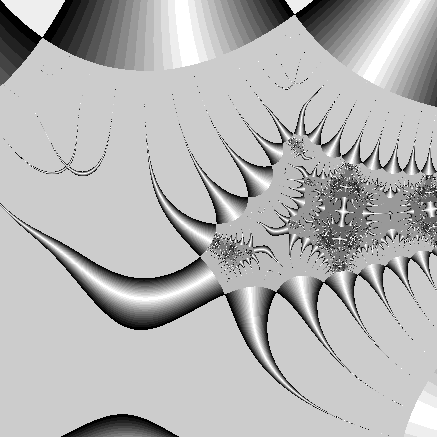The "c" in the above equations is a constant, a different form will be created for each value of "c", noting that some are more interesting than others.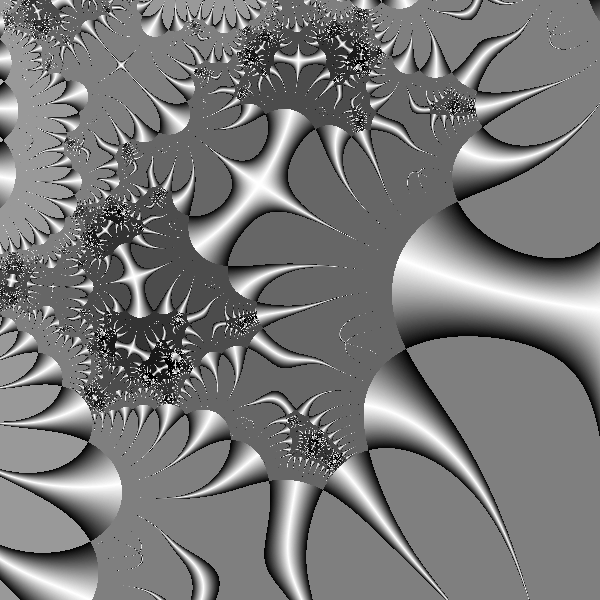zn+1 = sin(zn) + zn2 + c
c = 0.3 + 0.3i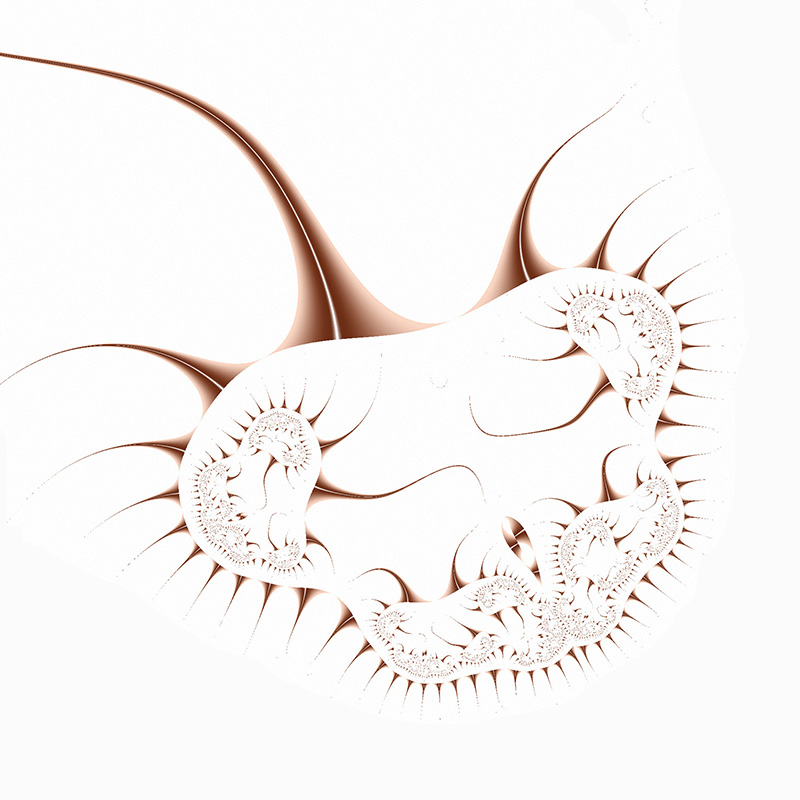zn+1 = znzn + zn5 + c
c = 0.5 - 0.1i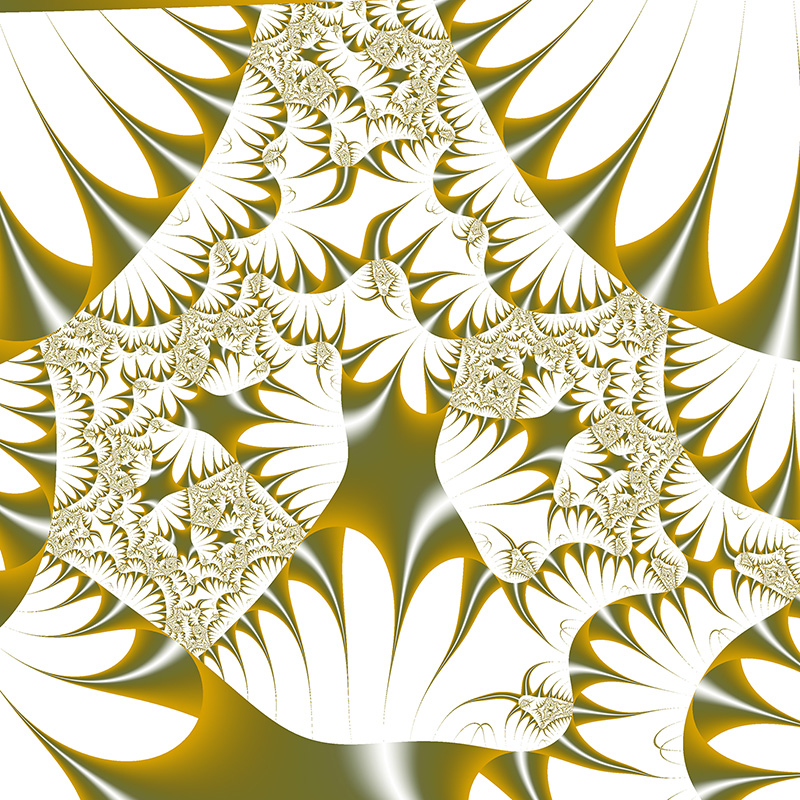zn+1 = sin(zn) + ezn + c
c = 0.1 + 0.6i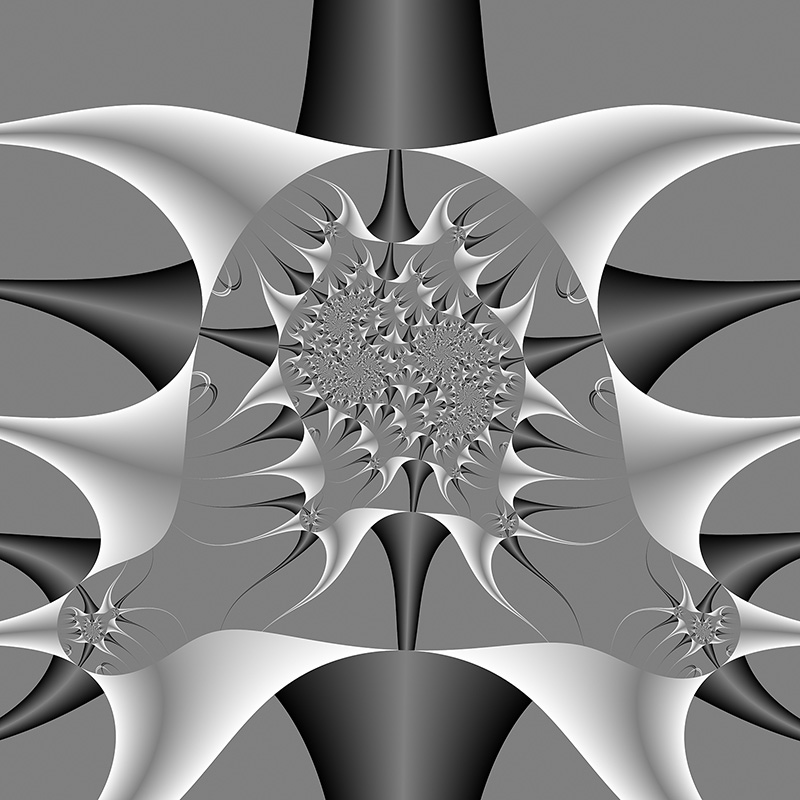zn+1 = sin(zn) + zn2 + c
c = 0.2 + 0.4i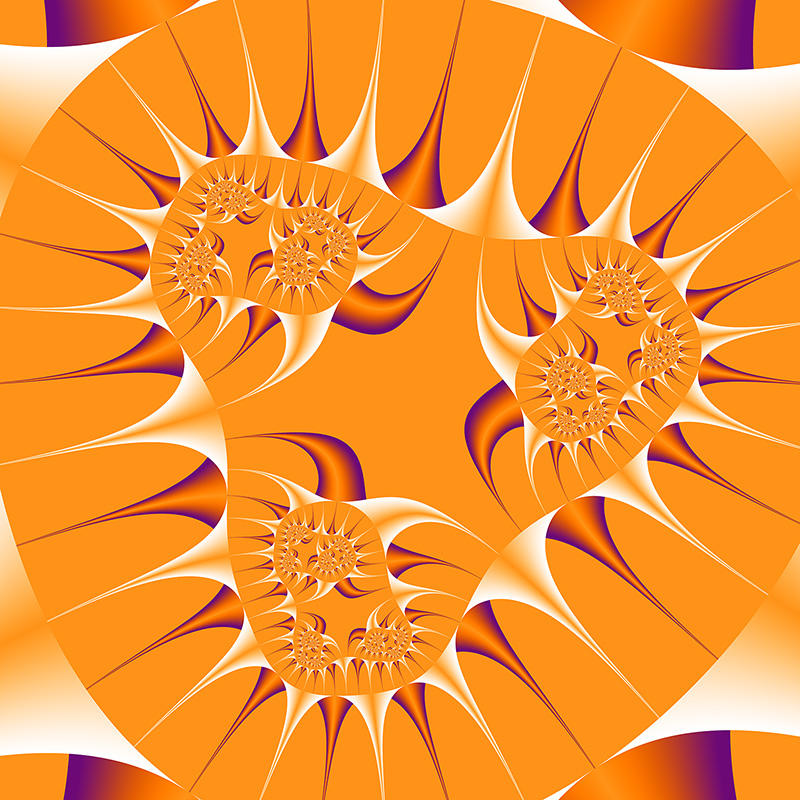zn+1 = zn3 + c
c = -1 + i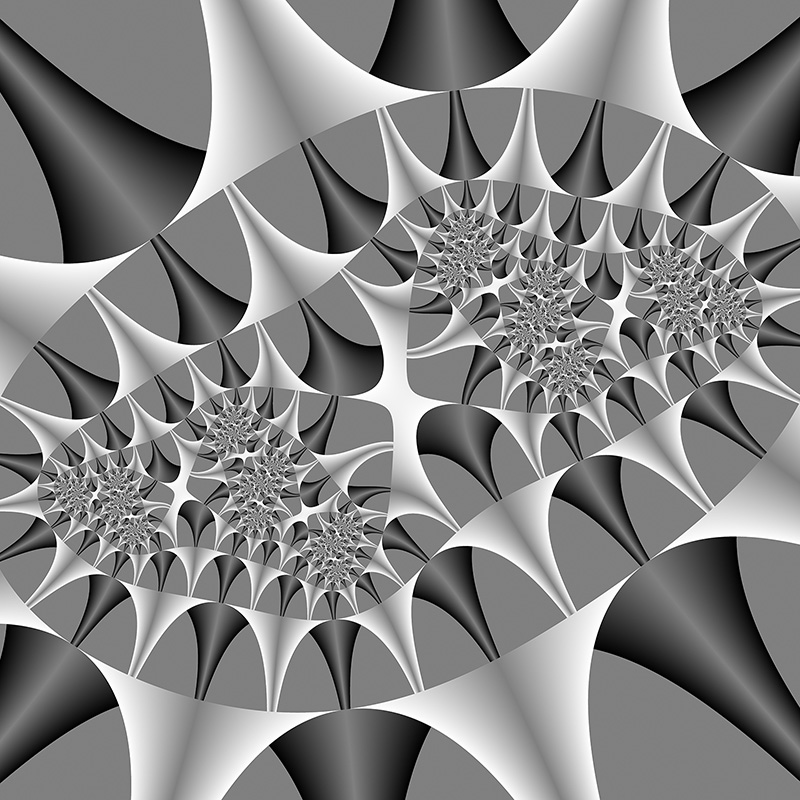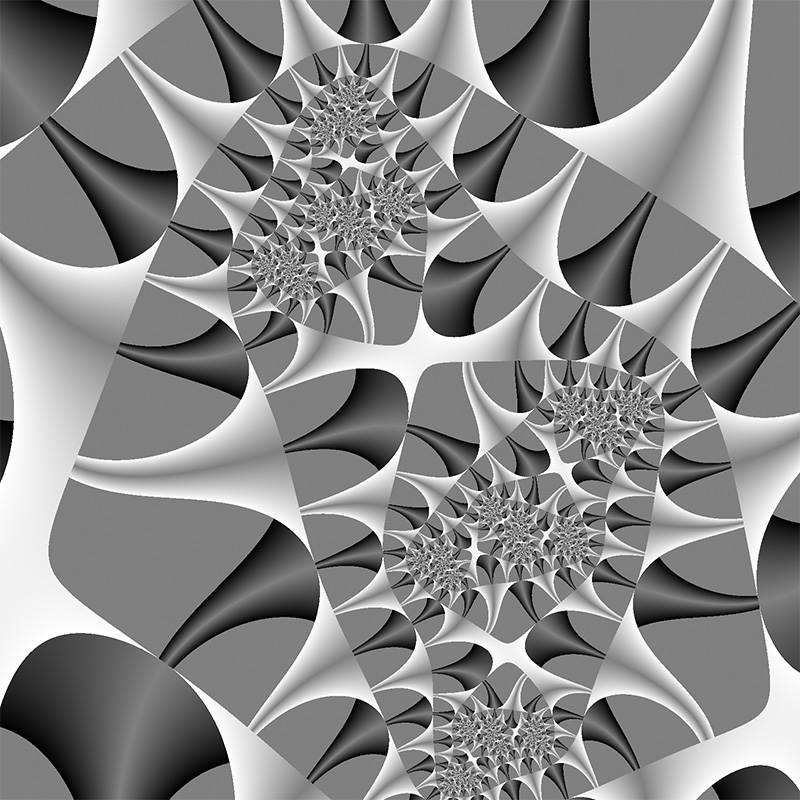zn+1 = zn2 + c
c = -1 + izn+1 = znzn + zn6 + c
c = 1 + izn+1 = zn5 + c
c = 1 + i